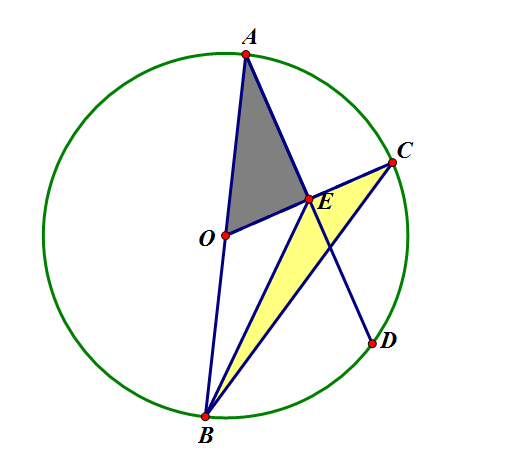# Find Area of Circle

Geometry Level 3$AB$ is the diameter of circle centered at $O$, $AD\bot OC$, $\triangle AOE$ and $\triangle CEB$ have the same area. The length of $AD$ is $1$, find the area of the circle.

×

Problem Loading...

Note Loading...

Set Loading...